# What is the molar mass of a gas that has a density of 2.62 g/L at...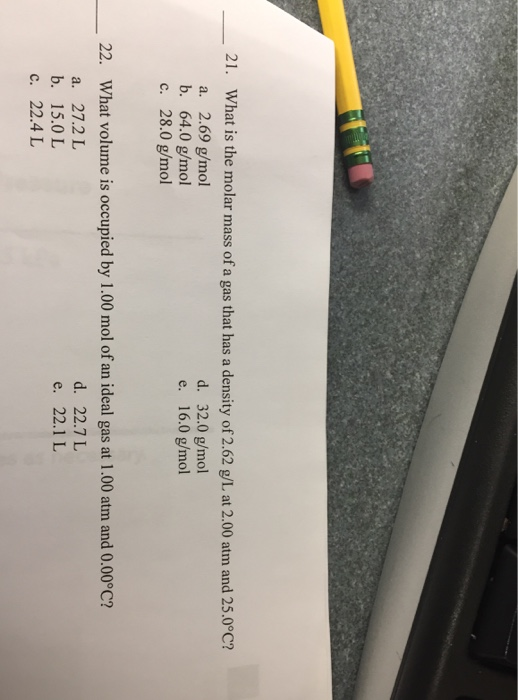What is the molar mass of a gas that has a density of 2.62 g/L at 2.00 atm and 25.0 degree C? 2.69 g/mol 64.0 g/mol 28.0 g/mol 32.0 g/mol 16.0 g/mol What volume is occupied by 1.00 mol of an ideal gas at 1.00 atm and 0.00degreeC? 27.2 L 15.0 L 22.4 L 22.7 L 22.1 L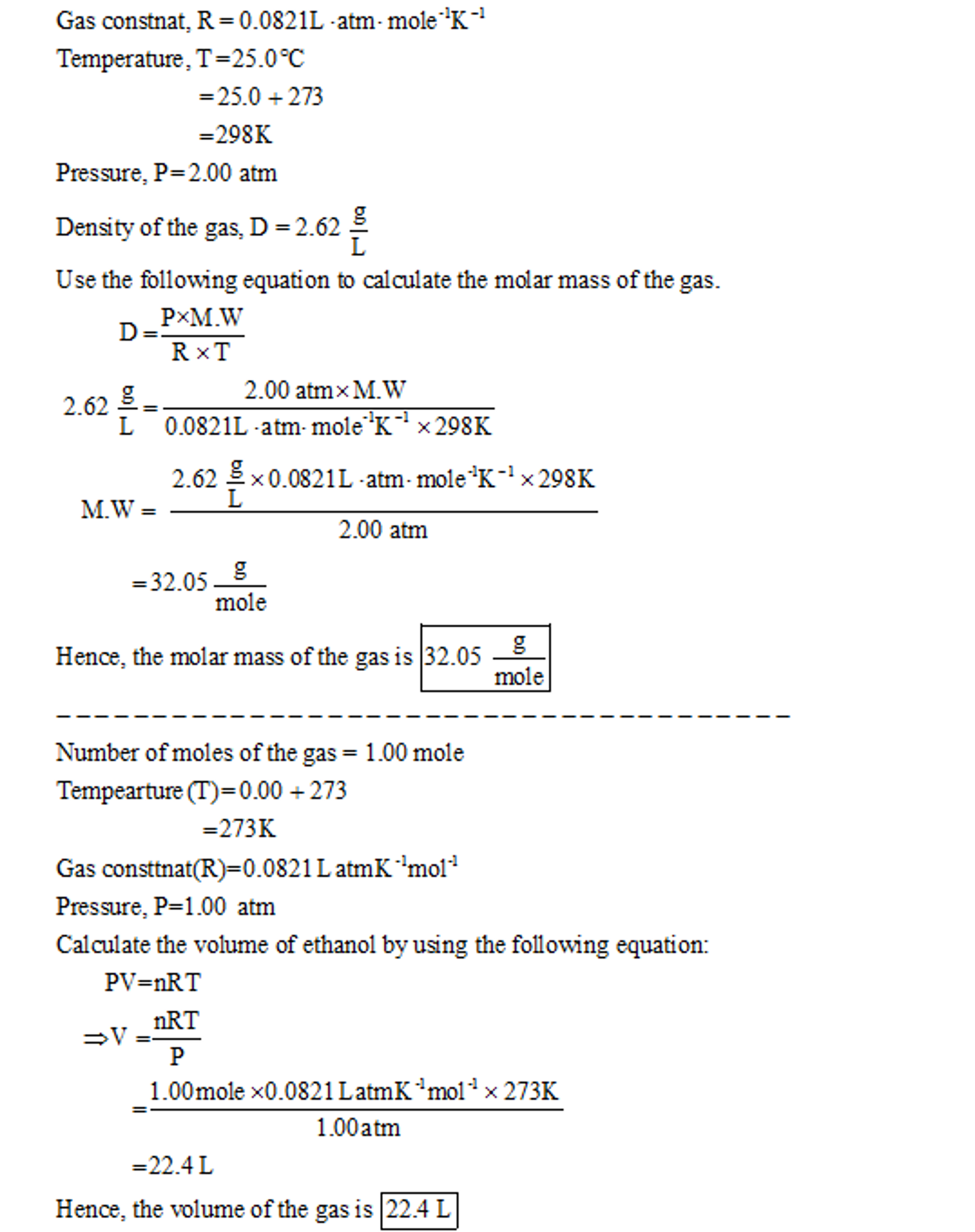#### Earn Coin

Coins can be redeemed for fabulous gifts.

Similar Homework Help Questions
• ### The density of a gas is 1.25 g/L at STP. What is its molar mass? A)...

The density of a gas is 1.25 g/L at STP. What is its molar mass? A) 22.4 g/mol B) 17.9 g/mol C) 28.0 g/mol D) 37.6 g/mol E) 41.6 g/mol please show work

• ### c. 64.0 g/mol 16. What is the temperature of Co, gas (density - 2.62 g/L) at...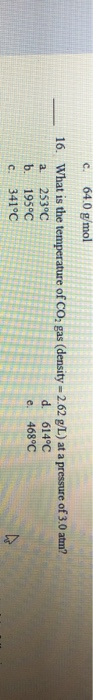c. 64.0 g/mol 16. What is the temperature of Co, gas (density - 2.62 g/L) at a pressure of 3.0 atm? a 253°C d. 614°C b. 195°C e. 468°C c. 341°C

• ### What is the molar mass of an unknown gas with a density of 2.50 g/L at...

What is the molar mass of an unknown gas with a density of 2.50 g/L at 1.00 atm and 25.0 °C?

• ### An unknown gas at 75.1 degree C and 1.00 atm has a molar mass of 30.07...

An unknown gas at 75.1 degree C and 1.00 atm has a molar mass of 30.07 g/mol. Assuming ideal behavior, what is the density of the gas? Answer __________ g/L

• ### What is the molar mass of an unknown gas with a density of 2.50 g/L at...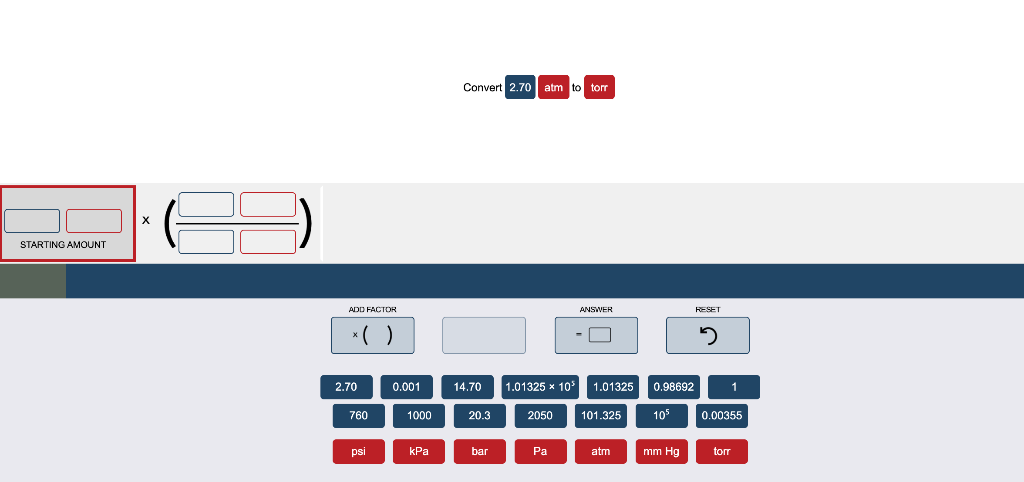What is the molar mass of an unknown gas with a density of 2.50 g/L at 1.00 atm and 25.0 °C? Convert 2.70 atm to torr STARTING AMOUNT ADD FACTOR ANSWER 0.98692 1 2.70 760 0.00114.70 1000 20.3 1.01325 * 10% 1.01325 2050 101.325 105 0.00355 psi | kPa bar Paatm mm Hgtor

• ### An unknown gas at 75.1 ∘C and 1.00 atm has a molar mass of 30.07 g/mol....

An unknown gas at 75.1 ∘C and 1.00 atm has a molar mass of 30.07 g/mol. Assuming ideal behavior, what is the density of the gas? density: ______ g/L

• ### The density of a gas is 0.803 g/L at STP. What is its molar mass? PICK...

The density of a gas is 0.803 g/L at STP. What is its molar mass? PICK ONE A 22.4 g/mol B 27.9 g/mol C 18.0 g/mol D 24.1 g/mol E 26.7 g/mol

• ### If the density of an unknown gas is 1.95 g/L at STP what is the molar...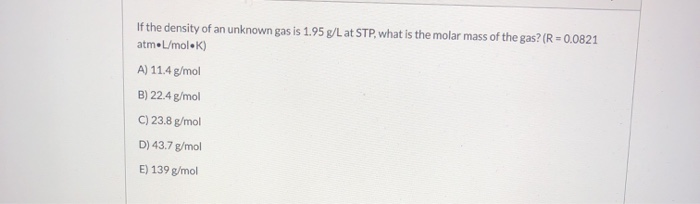If the density of an unknown gas is 1.95 g/L at STP what is the molar mass of the gas? (R = 0.0821 atmL/molek) A) 11.4 g/mol B) 22.4 g/mol C) 23.8 g/mol D) 43.7 g/mol E) 139 g/mol

• ### An unknown gas at 59.1 °C and 1.00 atm has a molar mass of 28.01 g/mol....

An unknown gas at 59.1 °C and 1.00 atm has a molar mass of 28.01 g/mol. Assuming ideal behavior, what is the density of the gas?

• ### What is the density (in g/L) of a gas with a molar mass of 122.7 g/mol at a pressure of 2.07 atm and a temperature of 52...

What is the density (in g/L) of a gas with a molar mass of 122.7 g/mol at a pressure of 2.07 atm and a temperature of 52.39 °C?

Free Homework App